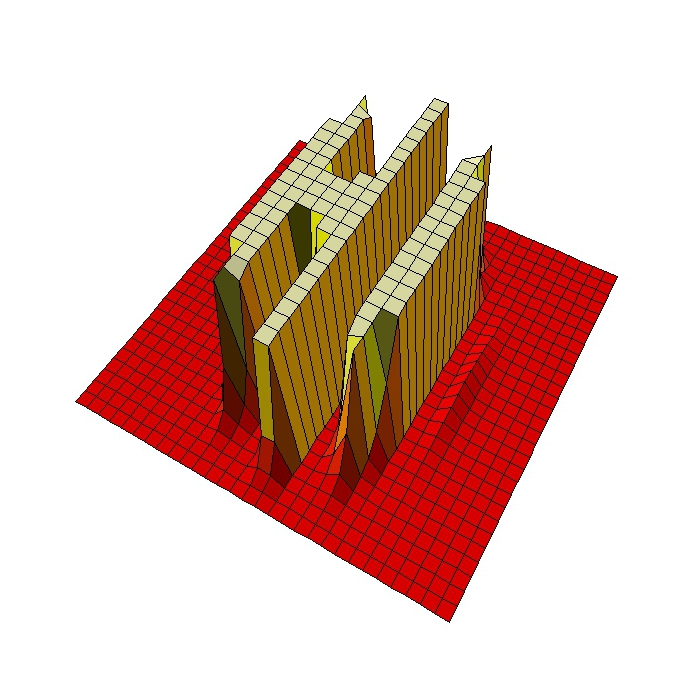# Hi

Why do some mathematicians wear a white coat? Are they afraid to be splashed by an integral? (Read on Twitter)

If you run into someone wearing a white coat who tells you something like

e raised to minus 3 by zero point five plus x squared plus y squared between two plus e raised to minus x squared minus y squared between two by cosine of four by x

do not be afraid: is just a harmless mathematician waving to you. Look at this:This is the code to draw these mathematical greetings:

```levelpersp=function(x, y, z, colors=heat.colors, ...) {
## getting the value of the midpoint
zz=(z[-1,-1] + z[-1,-ncol(z)] + z[-nrow(z),-1] + z[-nrow(z),-ncol(z)])/4
## calculating the breaks
breaks=hist(zz, plot=FALSE)\$breaks
## cutting up zz
cols=colors(length(breaks)-1)
zzz=cut(zz, breaks=breaks, labels=cols)
## plotting
persp(x, y, z, col=as.character(zzz), ...)
}
x=seq(-5, 5, length= 30);y=x
f=function(x,y) {exp(-3*((0.5+x)^2+y^2/2))+exp(-x^2-y^2/2)*cos(4*x)}
z=outer(x, y, f)
z[z>.001]=.001;z[z<0]=z[1,1]
levelpersp(x, y, z, theta = 30, phi = 55, expand = 0.5, axes=FALSE, box=FALSE, shade=.25)
```